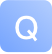数据结构与算法时间复杂度的问题-问答-阿里云开发者社区-阿里云数据结构与算法时间复杂度的问题

2018-07-21 16:18:44 975 1
Tn=n+(n+1)+(n-4)(2n+1)+2n(n-1)=4n^2-2n-1为什么若用O数量级估计，则有T（n）=O（n^2）取消 提交回答

• 小哇
2019-07-17 22:53:13
首先要理解几个概念：

【概念】
Tn ：一个算法中的语句执行次数称为语句频度或时间频度，记为T(n)。n称为问题的规模，当n不断变化时，时间频度T(n)也会不断变化。
* 算法中基本操作重复执行的次数，是问题规模 n 的某个函数，用T(n)表示

O(f(n))：称算法的渐进时间复杂度。
* 若有某个辅助函数f(n),使得当n趋近于无穷大时，T(n)/f(n)的极限值为不等于零的常数，则称f(n)是T(n)的同数量级函数记作T(n)=O(f(n))
*T(n)=O(f(n))，这里的"O"是数学符号，它的严格定义是 "若T(n)和f(n)是定义在正整数集合上的两个函数，则T(n)=O(f(n))表示存在正常数C和n0 ,使得当n≥n0时都满足0≤T(n)≤C*f(n)。"（即书本中的定义）。通俗一点就是这两个函数当整型自变量n趋向于无穷大时，两者的比值是一个不等于0的常数。

【分析】
T(n)是某个算法的时间耗费，它是该算法所求解 问题规模 n的函数，而后者O(f(n))是指当问题规模趋向无穷大时，该算法时间复杂度的数量级。当我们评价一个算法的时间性能时，主要标准就是算法的渐近时间复杂度O(f(n))，因此，在算法分析时，往往对两者不予区分，经常是将渐近时间复杂度T(n)=O(f(n))简称为时间复杂度，其中的f(n)一般是算法中频度最大的语句频度。

【计算】
Tn=n+(n+1)+(n-4)(2n+1)+2n(n-1)=4n^2-2n-1
当n→∞时 , T(n),f(n^2) 两个函数的比值是一个常数。
所以这个关系成立：T(n)=O(n^2)

【常见的时间复杂度】
按数量级递增排列依次为：
常数阶O(1)、对数阶O(log2n)或O(lbn)、线性阶O(n)、线性对数阶O(n*log2n)、平方阶O(n^2)、立方阶O(n^3)、k次方阶O(n^k)、指数阶O(2^n)。
0 0

1

25

qilu 2014-05-20 18:13:19 30190浏览量 回答数 25

38

xiaofanqie 2014-12-25 15:13:38 92130浏览量 回答数 38

9

jagen 2014-07-23 14:00:21 25247浏览量 回答数 9

37

13

【阿里云产品公测】开放搜索服务之 智能聊天实现

6

d1004 2011-11-23 14:35:13 24704浏览量 回答数 6

26

24

5

C语言算法 【精品问答合集】

10077

2994

《2021云上架构与运维峰会演讲合集》

《零基础CSS入门教程》

《零基础HTML入门教程》